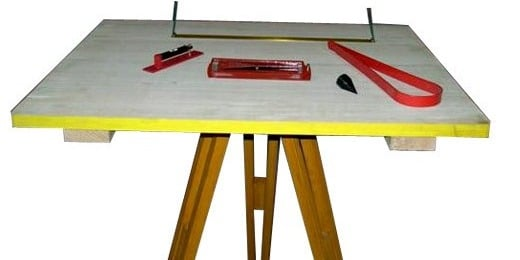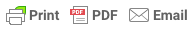Sorry, you do not have permission to ask a question, You must login to ask question. Become VIP Member

Meanings of various terms used in plane table surveying must be known before attempting for survey. Each term in plane table survey is useful and their determination during the surveying procedure is important.

## Terms Used in Plane Table Surveying

### 1. Centering

Centering is the process of setting up plane table in such a way that the plotted position ‘o’ on the drawing sheet corresponding to the ground station ‘O’ is exactly over the station.

### 2. Orientation

It is the alignment of plane table by rotating it in the horizontal plane so that all the plotted lines are parallel to the corresponding ground lines. It is done by using a trough compass by back sighting or resection.

### 3. Back Sight

Back sight is taken from the plane table station to another station whose position is known and is already been plotted on the drawing sheet. It is a method of orientation of plane table.

To back sight a station, let’s say A, when the plane is centered over the station A, the alidade is placed along the plotted line ab. Plane table is then rotated till the station B is bisected. Thus, plane table is oriented by back sighting.### 4. Fore Sight

Fore sight is taken from the plane table station to another station whose position has not been marked on the drawing sheet.

It is the method of locating a point by drawing a radial line from the plane table station to the station under consideration. For this, the plane table is set up and orientation. Then a ray is drawn in the direction of that point using alidade. A length equal to distance of that point is cut off to a suitable scale.& Ad Free!

GET VIP MEMBERSHIP NOW!

### 6. Intersection

Intersection in plane table surveying is the method of location the point by intersection of two rays drawn from two different stations. It is used when it is not possible, or it is difficult to measure the radial distance of the unknown point due to some obstruction and the radiation method cannot be used.

### 7. Resection

Resection in plane table survey is the method of locating a station occupied by the plane table when the position of that station has not been earlier plotted on the drawing sheet when the plane table occupied other station.

Resection is done by sighting two points whose position has earlier been plotted in the two-point problem. Alternatively, it can be done by sighting three points whose positions have earlier been plotted in the three-point problem.

### 8. Plane table traversing

It is a method of traversing the plane table during survey. The traverse is directly plotted on the drawing sheet by drawing traverse lines with the help of an alidade. No angle measurements are during plane table traversing.

Terms Used in Leveling and their Uses in Surveying

Plane Table Surveying with Methods and Examples

Equipments for Plane Table Survey and Their Uses